Check the below Online Education NCERT MCQ Questions for Class 6 Maths Chapter 10 Mensuration with Answers Pdf free download. MCQ Questions for Class 6 Maths with Answers were prepared based on the latest exam pattern. We have provided Mensuration Class 6 Maths MCQs Questions with Answers to help students understand the concept very well.

Students can also refer to NCERT Solutions for Class 6 Maths Chapter 10 Mensuration for better exam preparation and score more marks.

## Online Education for Mensuration Class 6 MCQs Questions with Answers

Mensuration Class 6 MCQ Question 1.
Perimeter of a rectangle =
(a) Length × Breadth
(b) Length + Breadth
(c) 2 × (Length + Breadth)
(d) 2 × (Length × Breadth).

MCQ On Mensuration For Class 6 Question 2.
Perimeter of a square =
(a) 4 × Length of a side
(b) 2 × Length of a side
(c) 3 × Length of a side
(d) 6 × Length of a side.

MCQ On Perimeter And Area For Class 6 Question 3.
Perimeter of an equilateral triangle
(a) 2 × Length of a side
(b) 3 × Length of a side
(c) 4 × Length of a side
(d) 6 x Length of a side.

Mensuration MCQ Class 6 Question 4.
Area of a rectangle =
(a) Length × Breadth
(b) Length + Breadth
(c) 2 × (Length + Breadth)
(d) 2 × (Length × Breadth).

Class 6 Maths Chapter 10 MCQ Question 5.
Area of a square =
(a) side × side
(b) 4 × Length of a side
(c) 2 × Length of a side
(d) 6 × Length of a side.

MCQ Questions For Class 6 Maths Chapter 10 Question 6.
Perimeter of a regular pentagon =
(a) 4 × Length of a side
(b) 3 × Length of a side
(c) 6 × Length of a side
(d) 5 × Length of a side.

Class 6 Mensuration MCQ Question 7.
Perimeter of a regular hexagon =
(a) 3 × Length of a side
(b) 4 × Length of a side
(c) 5 × Length of a side
(d) 6 × Length of a side.

MCQ Of Mensuration Class 6 Question 8.
Apala went to a park 20 m long and 10 m wide. She took one complete round of it. The distance covered by her is
(a) 30 m
(b) 60 m
(c) 20 m
(d) 10 m.

Hint:
Distance covered = 2(20 + 10) = 60 m

Class 6 Maths Mensuration MCQ Question 9.
The perimeter of the figure is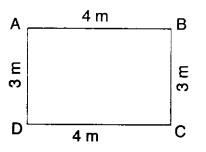(a) 12m
(b) 14m
(c) 24 m
(d) 7 m.

Hint:
Perimeter = 2(4 + 3) = 14m

Ncert Class 6 Maths Chapter 10 MCQ Question 10.
The perimeter of the figure is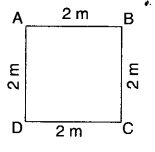(a) 8m
(b) 16m
(c) 4m
(d) none of these.

Hint:
Perimeter = 4 × 2 = 8m

MCQ On Area And Perimeter Class 6 Question 11.
A page is 25 cm long and 20 cm wide. Find the perimeter of this page.
(a) 90 cm
(b) 45 cm
(c) 500 cm
(d) 5 cm.

Hint:
Perimeter = 2(25 + 20) = 90 cm

Class 6 Maths Ch 10 MCQ Question 12.
The perimeter of the figure is(a) 5 cm
(b) 10 cm
(c) 15 cm
(d) 20 cm.

Hint:
Perimeter = 4(1 + 2 + 2) = 20cm

MCQ Class 6 Maths Chapter 10 Question 13.
The perimeterof the figure is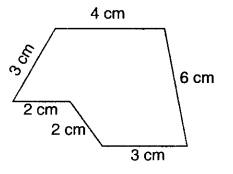(a) 20 cm
(b) 10 cm
(c) 24 cm
(d) 15 cm.

Hint:
Perimeter = 4 + 3 + 2 + 2 + 3 + 6 = 20 cm

Class 6 Mensuration MCQs Question 14.
Meenu wants to put a lace border all around a rectangle table cover 2 m long and 1 m wide. Find the length of the lace required by Meenu.
(a) 3 m
(b) 4 m
(c) 5 m
(d) 6m.

Hint:
Length of the lace = 2(2 + 1) = 6 m

MCQ Mensuration Class 6 Question 15.
Find the perimeter of a rectangle whose length and breadth are 9 cm and 1 cm respectively,
(a) 10 cm
(b) 20 cm
(c) 30 cm
(d) 40 cm.

Hint:
Perimeter = 2(9 + 1) = 20 cm

Question 16.
An athlete takes 10 rounds of a rectangular park, 40 m long and 30 m wide. Find the total distance covered by him.
(a) 1400 m
(b) 700 m
(c) 70 m
(d) 2800 m.

Hint:
Total distance covered = 10 × 2 × (40 + 30) = 1400 m

Question 17.
Find the cost of fencing a rectangular park of length 10 m and breadth 5 m at the rate of? 10 per metre.
(a) ₹ 300
(b) ₹ 600
(c) ₹ 150
(d) ₹ 1200.

Hint:
Cost= 10 × 2 × (10 + 5) = ₹ 300

Question 18.
The perimeter of a square of side 1 m is
(a) 1 cm
(b) 2 cm
(c) 3 cm
(d) 4 m.

Hint:
Perimeter = 4 × 1 =4m

Question 19.
The perimeter of an equilateral triangle of side 1 m is
(a) 1 m
(b) 2 m
(c) 3 m
(d) 6 m.

Hint:
Perimeter = 3 × 1 = 3 m

Question 20.
The perimeter of a regular pentagon of side 1 m is
(a) 5 m
(b) 10 m
(c) 15m
(d) 20m.

Hint:
Perimeter = 5 × 1 = 5 m

Question 21.
The perimeter of a regular hexagon of side 1 m is
(a) 3 m
(b) 2 m
(c) 4 m
(d) 6 m.

Hint:
Perimeter = 6 × 1 = 6m

Question 22.
Find the distance travelled by Sangeeta if she takes 5 rounds of a square park of side 10 m.
(a) 200 m
(b) 100 m
(c) 400m
(d) 800 m.

Hint:
Distance = 5 × 4 × 10 = 200 m

Question 23.
The perimeter of an equilateral triangle is 9 m. Find the length of the side.
(a) 1 m
(b) 2 m
(c) 3 m
(d) 9 m.

Hint:
Length of side = $$\frac { 9 }{ 3 }$$ = 3 m

Question 24.
The perimeter of a square is 8 m. Find the length of the side.
(a) 1m
(b) 2m
(c) 4m
(d) 8m.

Hint:
Length of side = $$\frac { 8 }{ 4 }$$ = 2 m

Question 25.
The perimeter of a regular pentagon is 10 m. Find the length of the side.
(a) 1m
(b) 2m
(c) 5m
(d) 10m

Hint:
Length of side = $$\frac { 10 }{ 5 }$$ = 2 m

Question 26.
The perimeter of a regular hexagon is 12 m. Find the length of the side.
(a) 2m
(b) 3m
(c) 4m
(d) 6m.

Hint:
Length of side = $$\frac { 12 }{ 6 }$$ = 2 m

Question 27.
The perimeter of the figure is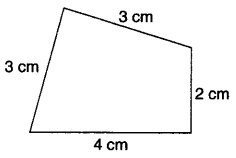(a) 12 cm
(b) 7 cm
(c) 6 cm
(d) 24 cm.

Hint:
Perimeter = 4 + 3 + 3 + 2 = 12 cm

Question 28.
The perimeter of the figure is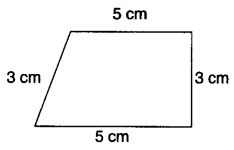(a) 16 cm
(b) 30 cm
(c) 7.5 cm
(d) 20 cm.

Hint:
Perimeter = 5 + 3 + 5 + 3 = 16 cm

Question 29.
The perimeter of the figure is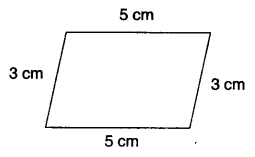(a) 8 cm
(b) 12 cm
(c) 15 cm
(d) 16 cm.

Hint:
Perimeter = 5 + 3 + 5 + 3 = 16 cm

Question 30.
The perimeter of the figure is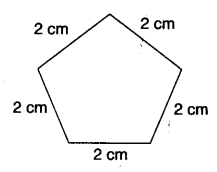(a) 10 cm
(b) 20 cm
(c) 15 cm
(d) 50 cm.

Hint:
Perimeter = 5 × 2 = 10 cm

Question 31.
The perimeter of a triangle of sides 2 cm, 3 cm and 4 cm is
(a) 9 cm
(b) 18 cm
(c) 27 cm
(d) 36 cm.

Hint:
Perimeter = 2 – 3 – 4 = 9cm

Question 32.
Two sides of a triangle are 5 cm and 4 cm. The perimeter of the triangle is 12 cm. The third side has length
(a) 1 cm
(b) 2 cm
(c) 3 cm
(d) 6 cm.

Hint:
Third side = 12 – (5 + 4) = 3 cm

Question 33.
A rectangular piece of land measures 0.5 km by 0.25 km. Each side is to be fenced with 4 rounds of wire. What is the length of the wire needed?
(a) 2 km
(b) 3 km
(c) 4km
(d) 6 km.

Hint:
Length of wire = 4 × 2 × (0.5 + 0.25) = 6 km.

Question 34.
The area of a rectangle of length 2 cm and breadth 1 cm is
(a) 1 cm2
(b) 2 cm2
(c) 4 cm2
(d) 8 cm2.

Hint:
Area = 2 × 1 = 2 cm2

Question 35.
The area of a square of side 1 cm is
(a) 1 cm2
(b) 4 cm2
(c) 9 cm2
(d) 16 cm2.

Hint:
Area = 1 × 1 = 1 cm2

Question 36.
The area of a rectangular sheet of paper is 20 cm2. Its length is 5 cm. Find its width.
(a) 1 cm
(b) 2 cm
(c) 3 cm
(d) 4 cm.

Hint:
Width = $$\frac { 20 }{ 5 }$$ = 4 cm

Question 37.
The perimeter of a rectangular piece of card board is 6 m. Its breadth is 1 m. Find its length.
(a) 1 m
(b) 2 m
(c) 3m
(d) 6m.

Hint:
Length = $$\frac { 6 }{ 2 }$$ – 1 = 2 m

Question 38.
The area of the figure is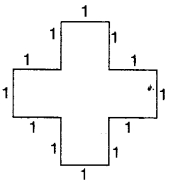(a) 1 sq. unit
(b) 5 sq. unit
(c) 4 sq. unit
(d) 6 sq. unit

Hint:
Area = 5 × 1 × 1 = 5

Question 39.
The area of the figure in (sq. unit) is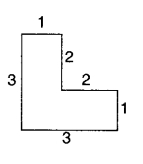(a) 1
(b) 5
(c) 4
(d) 6

Hint:
Area = 2 × 1 + 2 × 1 + 1 × 1 =5

Question 40.
The area of the figure is(a) 5 sq. unit
(b) 9 sq. unit
(c) 7 sq. unit
(d) 8 sq. unit.

Area = 3 × $$\frac { 1 }{ 2 }$$ + 1 × 2 + 3 × $$\frac { 1 }{ 2 }$$ = 5.